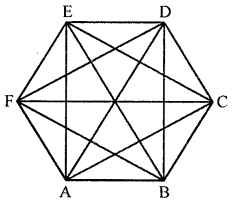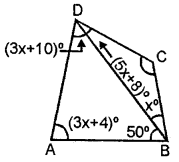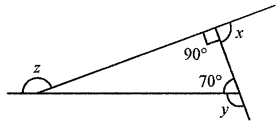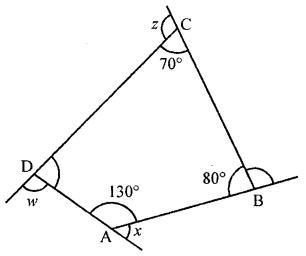## ML Aggarwal Class 8 Solutions for ICSE Maths Chapter 13 Understanding Quadrilaterals Ex 13.1

Question 1.
Some figures are given below.Classify each of them on the basis of the following:
(a) Simple curve
(b) Simple closed curve
(c) Polygon
(d) Convex polygon
(e) Concave polygon
Solution:
(a) (i), (ii), (iii), (v) and (vi) are simple curves.
(b) (iii), (v), (vi) are simple closed curves.
(c) (iii) and (vi) are polygons.
(d) (iii) is a convex polygon.
(e) (v) is a concave polygon.

Question 2.
How many diagonals does each of the following have?
(b) A regular hexagon
Solution:
(a) A convex quadrilateral: It has two diagonals.
(b) A regular hexagon: It has 9 diagonals as shown.Question 3.
Find the sum of measures of all interior angles of a polygon with number of sides:
(i) 8
(ii) 12
Solution:
(i) Sum of measures of all interior angles of
8-sided polygon = (2n – 4) × 90°
= (2 × 8 – 4) × 90°
= 12 × 90° = 1080°
(ii) Sum of measures of all interior angles of
12-sided polygon = (2n – 4) × 90°
= (2 × 12 – 4) × 90°
= 18 × 90°= 1800°

Question 4.
Find the number of sides of a regular polygon whose each exterior angles has a measure of
(i) 24°
(ii) 60°
(iii) 72°
Solution:
(i) Let number of sides of the polygon = n
Each exterior angle = 24°
∴ n = $$\frac{360^{\circ}}{24^{\circ}}$$ = 15 sides
∴ Polygon is of 15 sides.
(ii) Each interior angle of the polygon = 60°
Let number of sides of the polygon = n
∴ n = $$\frac{360^{\circ}}{60^{\circ}}$$ = 6
∴ Number of sides = 6
(iii) Each interior angle of the polygon = 72°
Let number of sides of the polygon = n
∴ n = $$\frac{360^{\circ}}{72^{\circ}}$$ = 5
∴ Number of sides = 5

Question 5.
Find the number of sides of a regular polygon if each of its interior angles is
(i) 90°
(ii) 108°
(iii) 165°
Solution:
(i) Each interior angle = 90°
Let number of sides of the regular polgyon = n
∴ 90° = $$\frac{2 n-4}{n}$$ × 90°
⇒ $$\frac{2 n-4}{n}=\frac{90^{\circ}}{90^{\circ}}$$ = 1
⇒ 2n – 4 = n
⇒ 2n – n = 4
⇒ n = 4
⇒ n = 4
∴ It is a square.
(ii) Each interior angle = 108°
Let number of sides of the regular polygon = n
∴ 108° = $$\frac{2 n-4}{n}$$ × 90°
⇒ $$\frac{2 n-4}{n}=\frac{108^{\circ}}{90^{\circ}}=\frac{6}{5}$$
⇒ 10n – 20 = 6n ⇒ 10n – 6n = 20
⇒ 4n = 20
⇒ n = $$\frac{20}{4}$$ = 5
∴ It is a pentagon.
(iii) Each interior angle = 165°
Let number of sides of the regular polygon = n
∴ 165° = $$\frac{2 n-4}{n}$$ × 90°
⇒ $$\frac{2 n-4}{n}=\frac{165^{\circ}}{90^{\circ}}=\frac{11}{6}$$
⇒ 12n – 24 = 11n
⇒ 12n – 11n = 24
⇒ n = 24
∴ It is 24-sided polygon.

Question 6.
Find the number of sides in a polygon if the sum of its interior angles is:
(i) 1260°
(ii) 1980°
(iii) 3420°
Solution:
We know that, sum of interior angles of polygon
is given by (2n – 4) at right angles.
(i) 1260°
∴ 1260 = (2n – 4) × 90
⇒ $$\frac{1260}{90}$$ = 2n – 4
⇒ 14 = 2n – 4
⇒ n = 9
(ii) 1980°
∴ 1980 = (2n – 4) × 90
⇒ $$\frac{1980}{90}$$ = 2n – 4
⇒ 22 = 2n – 4
⇒ n = 13.
(iii) 3420°
∴ 3420 = (272 – 4) × 90 3420
⇒ $$\frac{3420}{90}$$ = 2n – 4
⇒ 38 = 2n – 4
⇒ n = 21

Question 7.
If the angles of a pentagon are in the ratio 7 : 8 : 11 : 13 : 15, find the angles.
Solution:
Ratio in the angles of a polygon = 7 : 8 : 11 : 13 : 15
Sum of angles of a pentagon = (2n – 4) × 90°
= (2 × 5 – 4) × 90°
= 6 × 90° = 540°
Let the angles of the pentagon be
7x, 8x, 11x, 13x, 15x
∴ 7x + 8x + 11x + 13x + 15x = 540°
⇒ 54x = 540° ⇒ x = $$\frac{540^{\circ}}{54}$$ = 10°
∴ Angles are 7 × 10° = 70°, 8 × 10° = 80°,
11 × 10° = 110°, 13 × 10° = 130° and 15 × 10°= 150°
∴ Angles are 70°, 80°, 110°, 130° and 150°

Question 8.
The angles of a pentagon are x°, (x – 10)°, (x + 20)°, (2x – 44)° and (2x – 70°) Calculate x.
Solution:
Angles of a pentaon are x°, (x – 10)°, (x + 20)°,
(2x – 44)° and (2x – 70°)
But sum of angles of a pentagon
= (2n – 4) × 90°
= (2 × 5 – 4) × 90°
= 6 × 90° = 540°
∴ x + x – 10° + x + 20° + 2x – 44° + 2x – 70° = 540°
⇒ 7x – 104° = 540°
⇒ 7x = 540° + 104° = 644°
⇒ x = $$\frac{644^{\circ}}{7}$$ = 92°
∴ x = 92°

Question 9.
The exterior angles of a pentagon are in ratio 1 : 2 : 3 : 4 : 5. Find all the interior angles of the pentagon.
Solution:
Let the exterior angles of the pentagon are x, 2x, 3x, 4x and 5x.
We know that sum of exterior angles of polygon is 360°.
∴ x + 2x + 3x + 4x + 5x = 360°
⇒ 15x = 360°
⇒ x = $$\frac{360^{\circ}}{15}$$
⇒ x = 24°
∴ Exterior angles are 24°, 48°, 72°, 96°, 120°
Interior angles are 180° – 24°, 180° – 48°, 180° – 72°, 180° – 96°,
180° – 120° i.e. 156°, 132°, 108°, 84°, 60°.

Question 10.
In a quadrilateral ABCD, AB || DC. If ∠A : ∠D = 2:3 and ∠B : ∠C = ∠7 : 8, find the measure of each angle.
Solution:
As AB || CD∠A + ∠D = 180° and ∠B + ∠C = 180°
⇒ 2x + 3x = 180° and 7y + 8y = 180°
5x = 180° and 15y = 180°
x = 36° and y = 12°
∴ ∠A = 2 × 36 = 72°
and ∠D = 3 × 36 = 108°
∠B = 7y = 7 × 12 = 84°
and ∠C = 8y = 8 × 12 = 96°

Question 11.
(i) x
(ii) ∠DABSolution:
∴ ∠A + ∠B + ∠C + ∠D = 360°
⇒ (3x + 4) + (50 + x) + (5x + 8) + (3x + 10) = 360
⇒ 3x + 4 + 50 + x + 5x + 8 + 3x + 10 = 360°
⇒ 12x + 72 = 360°
⇒ 12x = 288
⇒ x = 24
(ii) ∠DAB = (3x + 4) = 3 × 24 + 4 = 76°
(iii) ∠ADB = 180°- (76° + 50°) = 54° (∵ ABD is a ∆)

Question 12.
Find the angle measure x in the following figures:Solution:
(i) In quadrilateral three angles are 40°, 140° and 100°But sum of Four angles = 360°
⇒ 40° + 140°+ 100° + x = 360°
⇒ 280° + x = 360°
⇒ x = 360° – 280° = 80°
(ii) In the given figure, ABCDE is a pentagon.
Where side AB is produced to both sides0∠1 + 60° = 180° (Linear pair)
∠1 = 180°- 60°= 120°
Similarly ∠2 + 80° = 180°
∴ ∠2 = 180°- 80°= 100°
Now, sum of angles of a pentagon = (2n – 4) × 90°
= (2 × 5 – 4) × 90° = 6 × 90° = 540°
∴ ∠A + ∠B + ∠C + ∠D + ∠E = 540°
⇒ 120° + 100° + x + 40° + x = 540°
⇒ 260° + 2x = 540°
⇒ 2x = 540° – 260° = 280°
⇒ x = $$\frac{280^{\circ}}{2}$$ = 140°
(iii) In the given figure, ABCD is a quadrilateral
whose side AB is produced is both sides ∠A = 90°But ∠A + ∠B + ∠C + ∠D = 360°
(Sum of angles of a quadrilateral)
⇒ 90°+ 60°+ 110° + x = 360°
⇒ 260° + x = 360°
⇒ x = 360° – 260° = 100°
∴ x = 100°
(iv) In the given figure, ABCD is a quadrilateral
whose side AB is produced to E.∠A = 90°, ∠C = 83°, ∠D = 110°
∠B + x = 180° (Lienar pair)
∠B = 180° – x
But ∠A + ∠B + ∠C + ∠D = 360°
⇒ 90° + (180° – x) + 83° + 110° = 360°
(Sum of angles of a quadrilateral)
⇒ 283°+ 180° – x = 360°
⇒ x = 283° + 180°- 360°
⇒ x = 463° – 360°= 103°

Question 13.
(i) In the given figure, find x + y + z.(ii) In the given figure, find x + y + z + w.Solution:
(i) In ∆ABC, Sides AB, BC, CA are produce in order in one side.∠B = 70°, ∠C = 90°
∴ ∠A = 180° – (∠B + ∠C)
= 180°- (70°+ 90°)
= 180°- 160° = 20°
But x + 90° = 180° (Linear pair)
∴ x = 180° – 90° = 90°
Similarly, y = 180° – 70° = 110°
y = 180° – 20°= 160°
x + y + z = 90° + 110°+ 160° = 360°
(ii) In the given figure,
ABCD is a quadrilateral whose sides are produced in order.∠A = 130°, ∠B = 80°, ∠C = 70°
∴ ∠D = 360° – (∠A + ∠B + ∠C)
= 360° – (130° + 80° + 70°)
= 360° – 280° = 80°
Now, x + 130° = 180° (Linear pair)
∴ x = 180°- 130° = 50°
Similarly, y = 180° – 80° = 100°
z = 180° – 70°= 110°
w = 180°-80°= 100°
∴ x + y + z + w = 52° + 100° +110°+ 100°
= 360°

Question 14.
A heptagon has three equal angles each of 120° and four equal angles. Find the size of equal angles.
Solution:
Sum of angles of a heptagon = (2 × n – 4) × 90°
= (2 × 7 – 4) × 90°
= 10 × 90° = 900°
Sum of three angles are each equal i.e. 120°
= 120° × 3 = 360°
Sum of remaining 4 equal angles
= 900° – 360° = 540°
∴ Each angle = $$\frac{540^{\circ}}{4}$$ = 135°

Question 15.
The ratio between an exterior angle and the interior angle of a regular polygon is 1 : 5. Find
(i) the measure of each exterior angle
(ii) the measure of each interior angle
(iii) the number of sides in the polygon.
Solution:
Ratio between an exterior and an interior angle = 1 : 5
Let exterior angle = x
Then interior angle = 5x
But sum of interior angle and exterior angle = 180°
∴ x + 5x = 180°
⇒ 6x = 180°
⇒ $$\frac{180^{\circ}}{6}$$ = 30°
(i) Measure of exterior angle x = 30°
(ii) and measure of interior angle = 5x = 5 × 30° = 150°
(iii) Let number of sides = n, then
$$\frac{2 n-4}{n}$$ × 90° = 150°
⇒ $$\frac{2 n-4}{n}=\frac{150^{\circ}}{90}=\frac{5}{3}$$
⇒ 6n – 12 = 5n
⇒ 6n – 5n = 12
⇒ n = 12
∴ Number of sides = 12

Question 16.
Each interior angle of a regular polygon is double of its exterior angle. Find the number of sides in the polygon.
Solution:
In a polygon,
Let exterior angle = x
Then interior angle = 2x
But sum of interior angle and exterior angle = 180°
∴ 2x + x = 180°
⇒ 3x = 180°
⇒ x = $$\frac{180^{\circ}}{3}$$ = 60°
∴ Interior angle = 2 × 60° = 120°
Let number of sides of the polygon = x
Then $$\frac{2 n-4}{n}$$ × 90° = 120°
⇒ $$\frac{2 n-4}{n}=\frac{120^{\circ}}{90}=\frac{4}{3}$$
⇒ 6n – 12 = 4n
⇒ 6n – 4n = 12
⇒ 2n = 12
⇒ n = $$\frac{12}{2}$$ = 6
∴ Number of sides = 6